# Titration: Acids and Bases 2. How can you determine which acid is diprotic? 3. using the...

Titration: Acids and Bases

2. How can you determine which acid is diprotic?

3. using the answers to questions one and two, which acid is diprotic?

4. Which base has more hydroxide ions per molecule?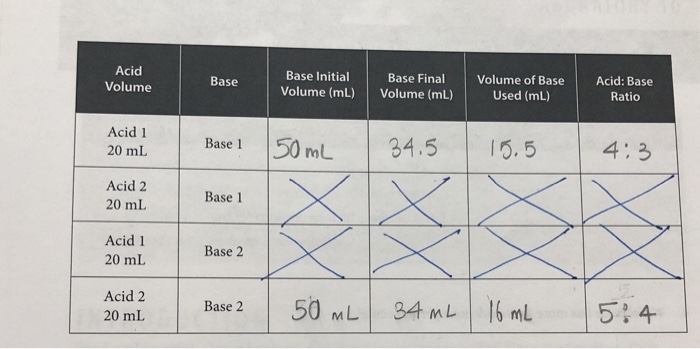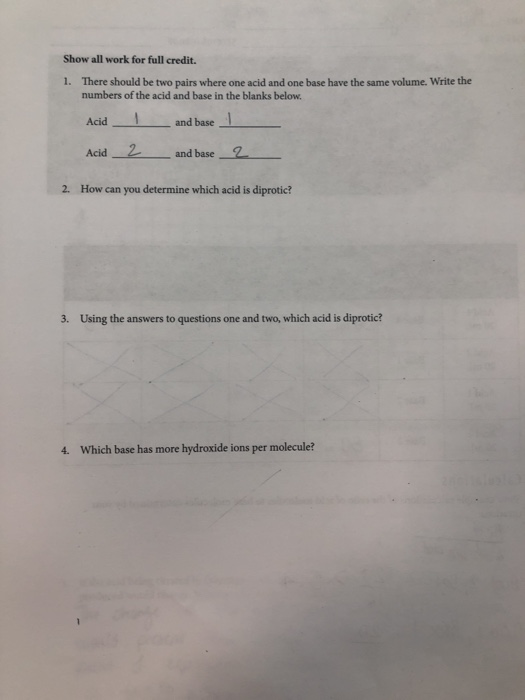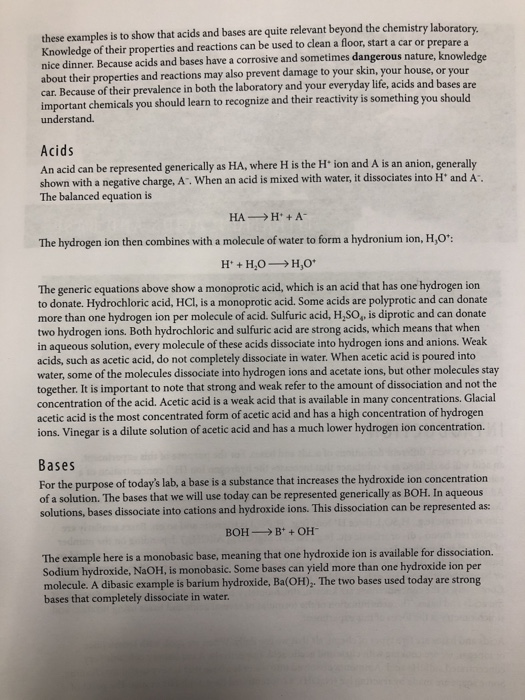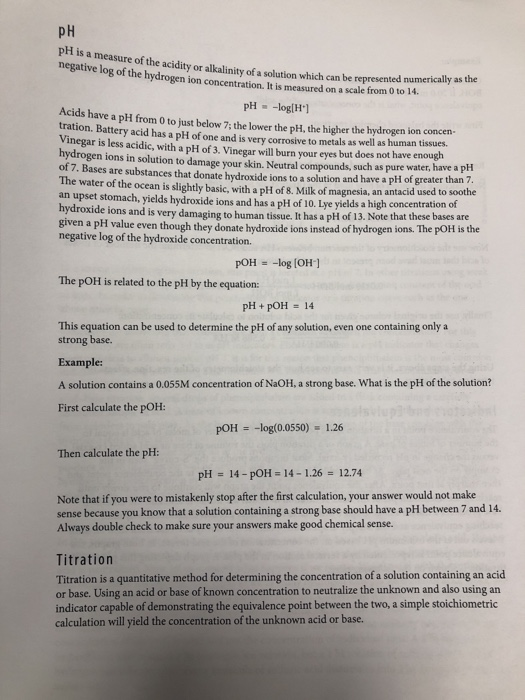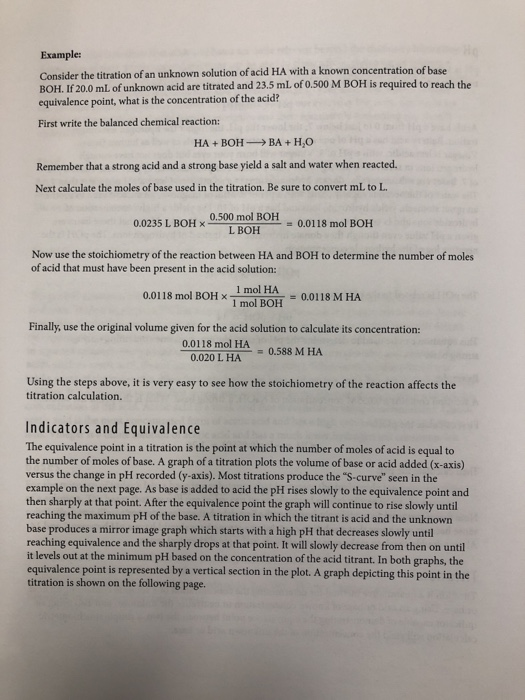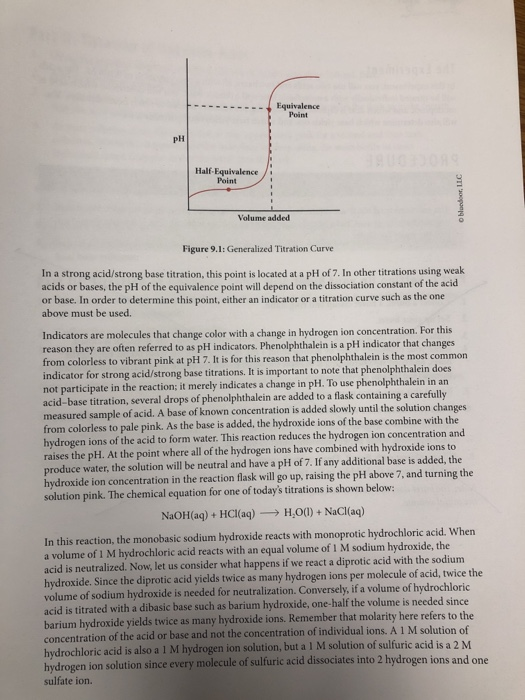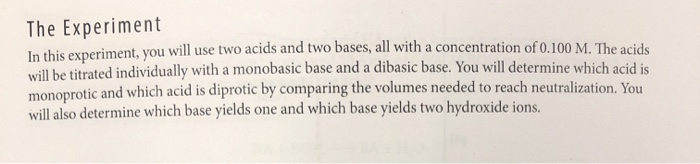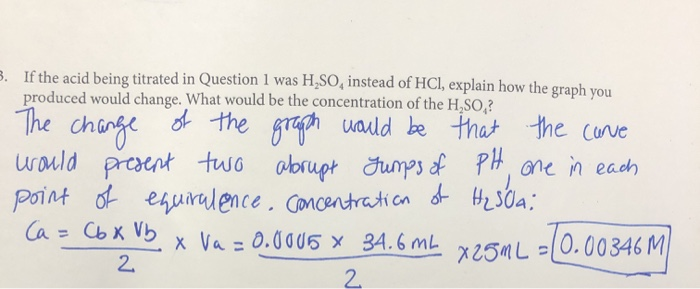Acid Volume Base Base Initial Volume (mL) Base Final Volume (mL) Volume of Base Used (mL) Acid: Base Ratio Acid 1 20 mL Base 1 50 mL 34.5 15.5 4:3 Acid 2 20 mL Base 1 Acid 1 20 mL Base 2 Acid 2 20 mL Base 2 50 mL 34 mL 16 mL 5:4
Show all work for full credit. 1. There should be two pairs where one acid and one base have the same volume. Write the numbers of the acid and base in the blanks below. Acidi Acid_2 and base | and base 2 2. How can you determine which acid is diprotic? 3. Using the answers to questions one and two, which acid is diprotic? 4. Which base has more hydroxide ions per molecule?
these examples is to show that acids and bases are quite relevant beyond the chemistry laboratory Knowledge of their properties and reactions can be used to clean a floor, start a car or prepare a nice dinner. Because acids and bases have a corrosive and sometimes dangerous nature, knowledge about their properties and reactions may also prevent damage to your skin, your house, or your car. Because of their prevalence in both the laboratory and your everyday life, acids and bases are important chemicals you should learn to recognize and their reactivity is something you should understand. Acids An acid can be represented generically as HA, where H is the Hion and A is an anion, generally shown with a negative charge. A. When an acid is mixed with water, it dissociates into Hand A. The balanced equation is HA -> H + A The hydrogen ion then combines with a molecule of water to form a hydronium ion, H,0": H +H, O H ,O* The generic equations above show a monoprotic acid, which is an acid that has one hydrogen ion to donate. Hydrochloric acid, HCl, is a monoprotic acid. Some acids are polyprotic and can donate more than one hydrogen ion per molecule of acid. Sulfuric acid, H,SO, is diprotic and can donate two hydrogen ions. Both hydrochloric and sulfuric acid are strong acids, which means that when in aqueous solution, every molecule of these acids dissociate into hydrogen ions and anions. Weak acids, such as acetic acid, do not completely dissociate in water. When acetic acid is poured into water, some of the molecules dissociate into hydrogen ions and acetate ions, but other molecules stay together. It is important to note that strong and weak refer to the amount of dissociation and not the concentration of the acid. Acetic acid is a weak acid that is available in many concentrations. Glacial acetic acid is the most concentrated form of acetic acid and has a high concentration of hydrogen ions. Vinegar is a dilute solution of acetic acid and has a much lower hydrogen ion concentration. Bases For the purpose of today's lab, a base is a substance that increases the hydroxide ion concentration of a solution. The bases that we will use today can be represented generically as BOH. In aqueous solutions, bases dissociate into cations and hydroxide ions. This dissociation can be represented as: BOH->B+ OH- The example here is a monobasic base, meaning that one hydroxide ion is available for dissociation. Sodium hydroxide, NaOH, is monobasic. Some bases can yield more than one hydroxide ion per molecule. A dibasic example is barium hydroxide, Ba(OH),. The two bases used today are strong bases that completely dissociate in water.
pH pH is a measure of the acidity or alkalinity of a solu negative log of the hydrogen ion concentration. It is measured on a scale from 0 to 14. acidity or alkalinity of a solution which can be represented numerically as the pH = -log[H] have a pH from 0 to just below 7, the lower the pH, the higher the hydrogen ion concen- tration. Battery acid has a pH of one and is very corrosive to metals as well as human tissues Vinegar is less acidic, with a pH of 3. Vinegar will burn your eyes but does not have enough hydrogen ions in solution to damage your skin. Neutral compounds, such as pure water, have a pH of 7. Bases are substances that donate hydroxide ions to a solution and have a pH of greater than The water of the ocean is slightly basic, with a pH of 8 Milk of magnesia, an antacid used to soothe an upset stomach, yields hydroxide ions and has a pH of 10. Lye yields a high concentration of hydroxide ions and is very damaging to human tissue. It has a pH of 13. Note that these bases are given a pH value even though they donate hydroxide ions instead of hydrogen ions. The port is the negative log of the hydroxide concentration. pOH = -log(OH) The pOH is related to the pH by the equation: pH + pOH = 14 This equation can be used to determine the pH of any solution, even one containing only a strong base. Example: A solution contains a 0.055M concentration of NaOH, a strong base. What is the pH of the solution? First calculate the pOH: pOH = -log(0.0550) - 1.26 Then calculate the pH: pH = 14 - pOH = 14 - 1.26 = 12.74 Note that if you were to mistakenly stop after the first calculation, your answer would not make sense because you know that a solution containing a strong base should have a pH between 7 and 14. Always double check to make sure your answers make good chemical sense. Titration Titration is a quantitative method for determining the concentration of a solution containing an acid or base. Using an acid or base of known concentration to neutralize the unknown and also using an indicator capable of demonstrating the equivalence point between the two, a simple stoichiometric calculation will yield the concentration of the unknown acid or base.
Example: Consider the titration of an unknown solution of acid HA with a known concentration of base BOH. If 20.0 mL of unknown acid are titrated and 23.5 mL of 0.500 M BOH is required to reach the equivalence point, what is the concentration of the acid? First write the balanced chemical reaction: HA+BOH BA + H,O Remember that a strong acid and a strong base yield a salt and water when reacted. Next calculate the moles of base used in the titration. Be sure to convert ml to L 0.0235 L BOH 0.500 mol BOH " -= 0.0118 mol BOH L BOH Now use the stoichiometry of the reaction between HA and BOH to determine the number of moles of acid that must have been present in the acid solution: 0.0118 mol BOH X mol BOH 1 mol HA = 0.0118 M HA Finally, use the original volume given for the acid solution to calculate its concentration: 0.0118 mol HA -= 0.588 M HA 0.020 L HA Using the steps above, it is very easy to see how the stoichiometry of the reaction affects the titration calculation. Indicators and Equivalence The equivalence point in a titration is the point at which the number of moles of acid is equal to the number of moles of base. A graph of a titration plots the volume of base or acid added (x-axis) versus the change in pH recorded (y-axis). Most titrations produce the "S-curve" seen in the example on the next page. As base is added to acid the pH rises slowly to the equivalence point and then sharply at that point. After the equivalence point the graph will continue to rise slowly until reaching the maximum pH of the base. A titration in which the titrant is acid and the unknown base produces a mirror image graph which starts with a high pH that decreases slowly until reaching equivalence and the sharply drops at that point. It will slowly decrease from then on until it levels out at the minimum pH based on the concentration of the acid titrant. In both graphs, the equivalence point is represented by a vertical section in the plot. A graph depicting this point in the titration is shown on the following page.
Equivalence Half-Equivalence Point blender Volume added Figure 9.1: Generalized Titration Curve In a strong acid/strong base titration, this point is located at a pH of 7. In other titrations using weak acids or bases, the pH of the equivalence point will depend on the dissociation constant of the acid or base. In order to determine this point, either an indicator or a titration curve such as the one above must be used. Indicators are molecules that change color with a change in hydrogen ion concentration. For this reason they are often referred to as pH indicators. Phenolphthalein is a pH indicator that changes from colorless to vibrant pink at pH 7. It is for this reason that phenolphthalein is the most common indicator for strong acid/strong base titrations. It is important to note that phenolphthalein does not participate in the reaction; it merely indicates a change in pH. To use phenolphthalein in an acid-base titration, several drops of phenolphthalein are added to a flask containing a carefully measured sample of acid. A base of known concentration is added slowly until the solution changes from colorless to pale pink. As the base is added, the hydroxide ions of the base combine with the hydrogen ions of the acid to form water. This reaction reduces the hydrogen ion concentration and raises the pH. At the point where all of the hydrogen ions have combined with hydroxide ions to produce water, the solution will be neutral and have a pH of 7. If any additional base is added, the hydroxide ion concentration in the reaction flask will go up, raising the pH above 7, and turning the solution pink. The chemical equation for one of today's titrations is shown below: NaOH(aq) + HCl(aq) -> H.O(l) + NaCl(aq) In this reaction, the monobasic sodium hydroxide reacts with monoprotic hydrochloric acid. When a volume of 1 M hydrochloric acid reacts with an equal volume of IM sodium hydroxide, the acid is neutralized. Now, let us consider what happens if we react a diprotic acid with the sodium hydroxide. Since the diprotic acid yields twice as many hydrogen ions per molecule of acid, twice the volume of sodium hydroxide is needed for neutralization. Conversely, if a volume of hydrochloric acid is titrated with a dibasic base such as barium hydroxide, one-half the volume is needed since barium hydroxide yields twice as many hydroxide ions. Remember that molarity here refers to the concentration of the acid or base and not the concentration of individual ions. A l M solution of hydrochloric acid is also a 1 M hydrogen ion solution, but a I M solution of sulfuric acid is a 2M hydrogen ion solution since every molecule of sulfuric acid dissociates into 2 hydrogen ions and one sulfate ion.
The Experiment In this experiment, you will use two acids and two bases, all with a concentration of 0.100 M. The acids will be titrated individually with a monobasic base and a dibasic base. You will determine which acid is monoprotic and which acid is diprotic by comparing the volumes needed to reach neutralization. You will also determine which base yields one and which base yields two hydroxide ions.
3. If the acid being titrated in Question 1 was H_SO, instead of HCl, explain how the graph you produced would change. What would be the concentration of the H.SO? The change of the graph would be that the care would present two abrupt Jumps of pH, one in each point of equirulence. Concentration of H₂SOA: Ca = 66 x Vb x Va = 0.0006 X 34.6 Mb x25ML -(0.00346 M 2

2.) By plotting the volume of base added vs pH curve we can predict if the given acid is monoprotic or diprotic .

If two sudden change in the pH is observed two times in the curve then the Acid is diprotic. Also we can do conductometric titrations and plot the conductivity vs volume of base added curve if the Two dips(points where conductivity start to increase or decrease) in the conductivity plot then diprotic acid otherwise monoprotic acid.

#### Earn Coin

Coins can be redeemed for fabulous gifts.

Similar Homework Help Questions
• ### 10.3 Titration curves are shown for two diprotic bases titrated with hydrochloric acid. Both bases are...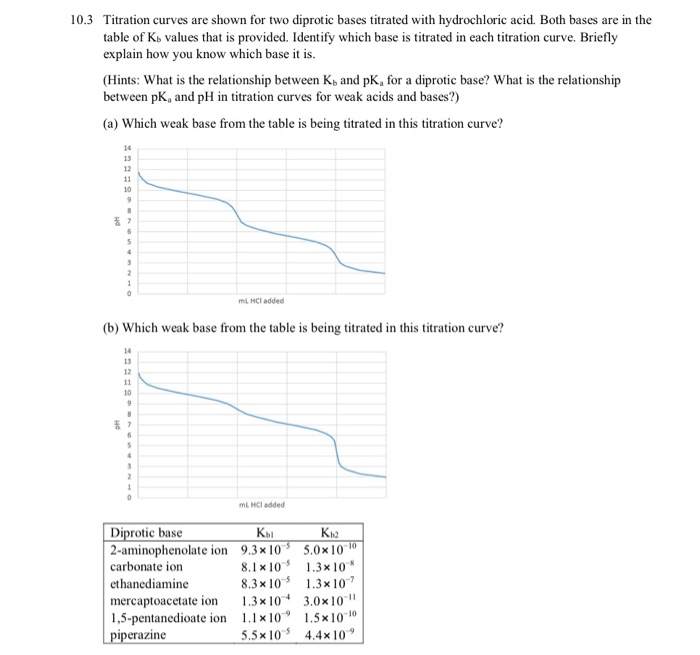10.3 Titration curves are shown for two diprotic bases titrated with hydrochloric acid. Both bases are in the table of Ko values that is provided. Identify which base is titrated in each titration curve. Briefly explain how you know which base it is. (Hints: What is the relationship between Ko and PK, for a diprotic base? What is the relationship between pK, and pH in titration curves for weak acids and bases?) (a) Which weak base from the table is...

• ### Acetic acid CH3COOH 1.8×10-5 Hypochlorous acid HClO 3.5×10-8 The titration of a weak acid with a...Acetic acid CH3COOH 1.8×10-5 Hypochlorous acid HClO 3.5×10-8 The titration of a weak acid with a strong base. In this assignment you will be asked to calculate the pH for various points in the titration of a weak acid with a strong base, where the reaction is of the form: HA + OH —— A+H2O (1) At the start of the titration before any strong base has been added. pH 12 10 8 At this point, the solution is that...

• ### Standardization of NaOH: Acid Base Titration Objective: In this lab, you will accurately determine the concentration...

Standardization of NaOH: Acid Base Titration Objective: In this lab, you will accurately determine the concentration of a solution of sodium hydroxide (NaOH) using a 0.500M potassium hydrogen phthalate (KHP) standard solution. Background: Acid–Base Titrations When an acid reacts with a base, a neutralization reaction occurs. The H+ ions from the acid and the HO– ions from the base combine to form water and are therefore neutralized. The other product of reaction is a salt. For example, hydrochloric acid reacts...

• ### Lab 5 Buffers 1. Dissolved ions in salt solutions can act as acids or bases and...

Lab 5 Buffers 1. Dissolved ions in salt solutions can act as acids or bases and react with water to produce hydronium ions or hydroxide ions that contribute to the pH of the salt solution. Since strong acids and strong bases completely ionize in solution, the reverse reaction essentially does not occur, meaning that the resulting conjugate base of a strong acid or conjugate acid of a strong base do NOT act as acids or bases. Ions that are conjugate...

• ### Concepts of Acids, Bases, and Conjugates 1) Rank the strengths of phosphoric acid, sulfuric acid and...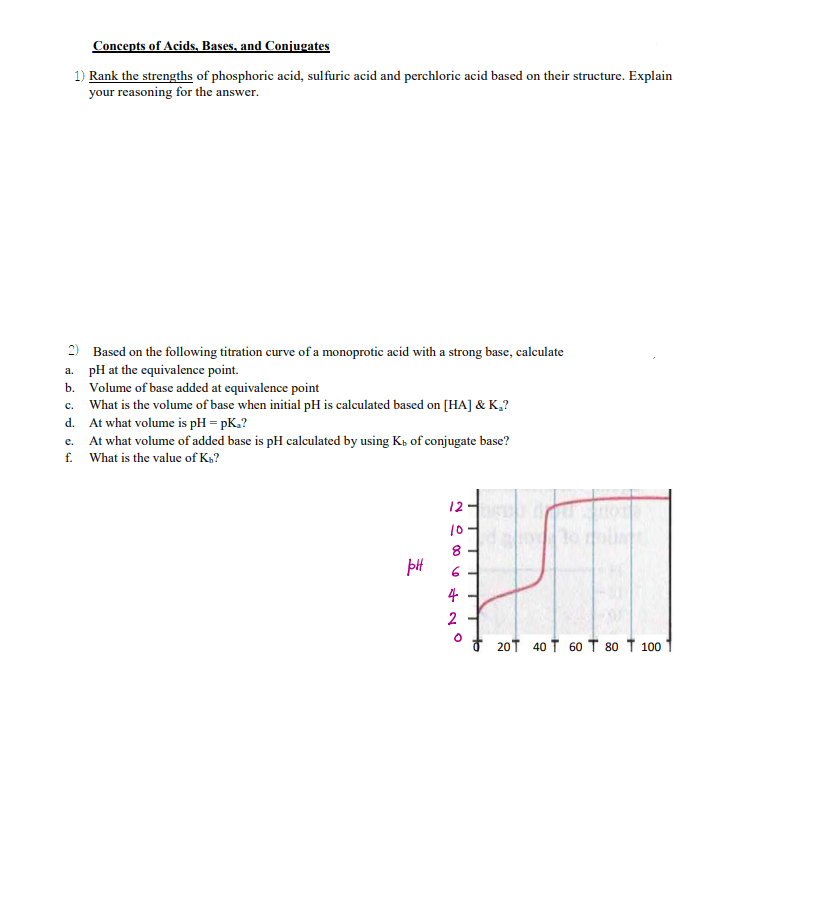Concepts of Acids, Bases, and Conjugates 1) Rank the strengths of phosphoric acid, sulfuric acid and perchloric acid based on their structure. Explain your reasoning for the answer. 2) Based on the following titration curve of a monoprotic acid with a strong base, calculate a. pH at the equivalence point. b. Volume of base added at equivalence point c. What is the volume of base when initial pH is calculated based on [HA] & K.? At what volume is pH...

• ### Homework Acids and Bases Name: 1) Write in the products for this acid-base neutralization reaction, then...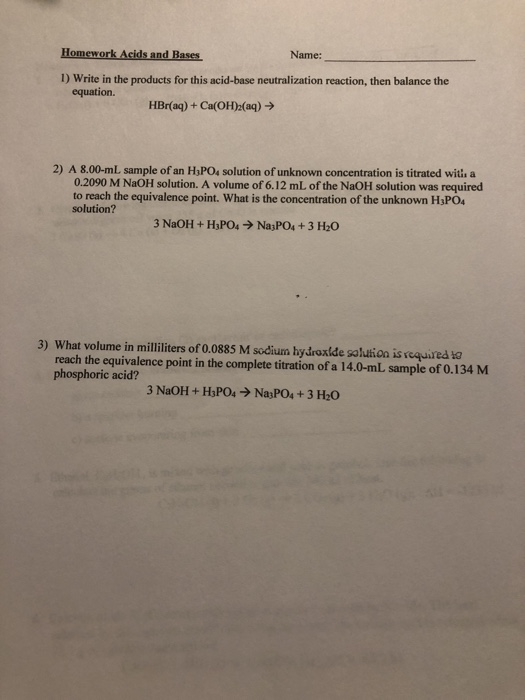Homework Acids and Bases Name: 1) Write in the products for this acid-base neutralization reaction, then balance the equation. HBr(aq) + Ca(OH)2(aq) → 2) A 8.00-ml sample of an H.PO, solution of unknown concentration is titrated with a 0.2090 M NaOH solution. A volume of 6.12 mL of the NaOH solution was required to reach the equivalence point. What is the concentration of the unknown H3PO4 solution? 3 NaOH + H3PO4 → Na3PO4 + 3 H20 3) What volume in...

• ### 7. If the second equivalence point in the titration of a diprotic acid is at 39.48...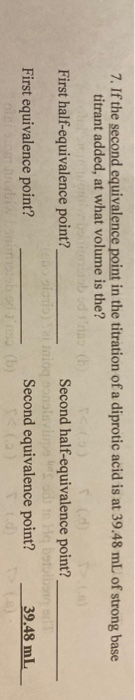7. If the second equivalence point in the titration of a diprotic acid is at 39.48 mL of strong base titrant added, at what volume is the? robudno b First half-equivalence point? Second half-equivalence point? iniog solving First equivalence point? Second equivalence point? 39.48 mL

• ### Titration of a diprotic acid with a strong base You have a 10.0 mL solution containing...

Titration of a diprotic acid with a strong base You have a 10.0 mL solution containing 0.5 M carbonic acid. Carbonic acid is diprotic, with pKa1 = 6.35 and pKa2 = 10.33. You titrate this solution using 1.00 M NaOH . (a) Calculate the pH of the solution before adding any NaOH. (b) Calculate the amount of NaOH needed to reach the first midpoint. What is the pH? (c) Calculate the amount of NaOH needed to reach the first equivalence...

• ### To learn about titration types and how to calculate pH at different points of titration. In an acid-base titration, a...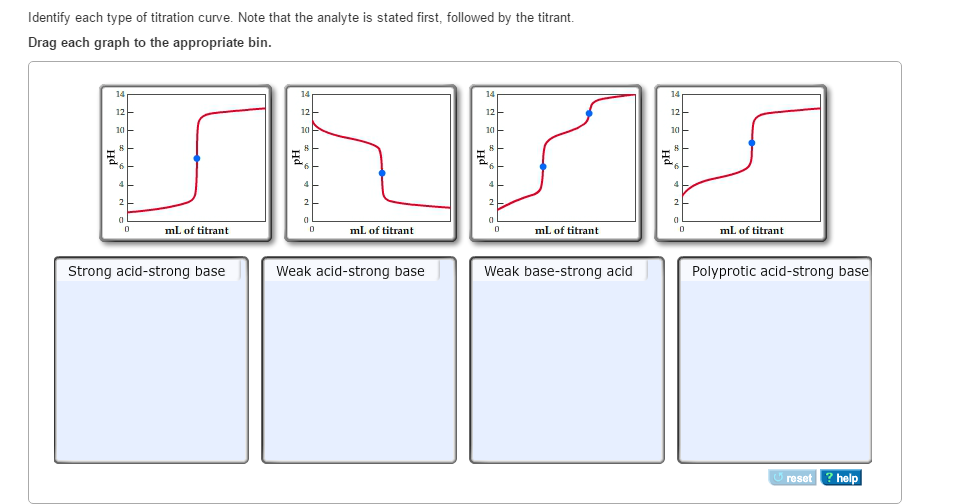To learn about titration types and how to calculate pH at different points of titration. In an acid-base titration, a titrant (solution of a base or acid) is added slowly to an analyte (solution of an acid or base). The titration is often monitored using a pH meter. A plot of pH as a function of the volume of titrant added is called a pH titration curve. Prior to the titration, the pH is determined by the concentration of the...

• ### 6. Titration of a 50.00 mL solution of an unknown diprotic acid required 35.95 mL of...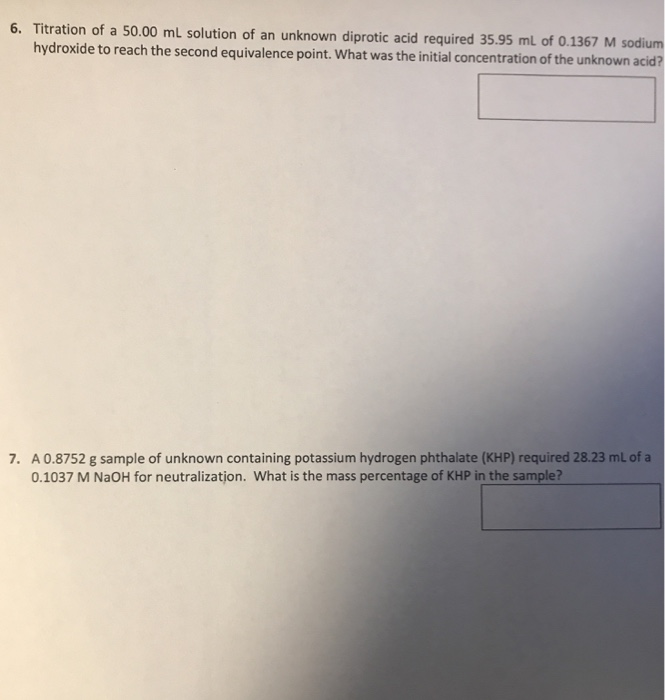6. Titration of a 50.00 mL solution of an unknown diprotic acid required 35.95 mL of 0.1367 M sodium hydroxide to reach the second equivalence point. What was the initial concentration of the unknown acid . A 0.8752 g sample of unknown containing potassium hydrogen phthalate (KHP) required 28.23 mL of a 0.1037 M NaOH for neutralizatjon. What is the mass percentage of KHP in the sample?